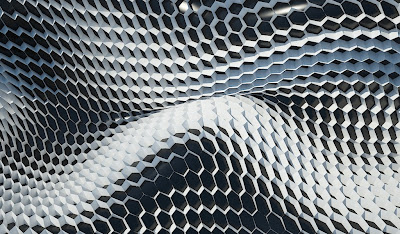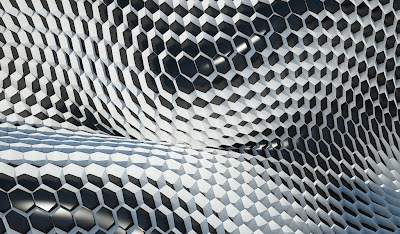## 27 April 2009

### Phyllotaxis system01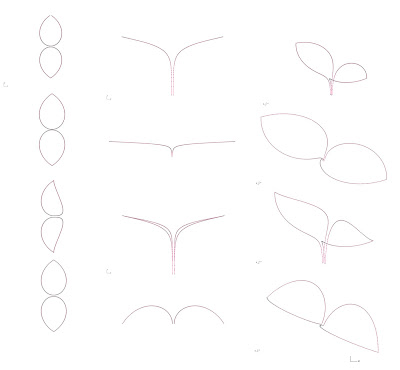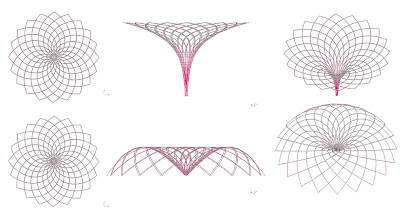From Wikipedia "In botany, phyllotaxis or phyllotaxy is the arrangement of the leaves on the stem of a plant.

I'm working on phyllotaxis system in order to construct vertical spiral variations with a rhinoscript code:

-plot an array of points by x,y,z coordinates follwing mathematical logic with several variations
-draws 2 lines in order to draw first petal profile
-divide profile in one array of points defined by the user
-draw circle with previous array points as centerpoint and curvature value of profile petal as radius
-create loft surfaces
-make a polar array to realize final system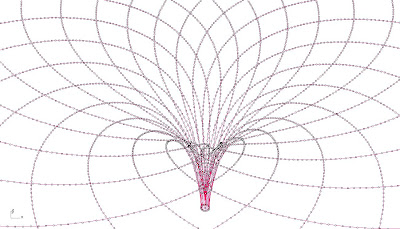"hot" code:
----------------------
'Script version Thursday, 16 April 2009 17:43:39

Call phyllotaxis_system()
Sub phyllotaxis_system()

Dim x,y,z,theta
Dim n:n=0

Dim dbla,dblb,dble,strobjects,strtxt,strline,ArrCurves3,p
'Call Rhino.EnableRedraw(False)

For n=0 To 50 Step 0.5
ReDim Preserve arrPts(n),arrPts1(n)
ReDim Preserve arrcircle(n),arrcone(n)
Dim arrplane
theta=(n/100)*rhino.pi
dbla=1
dblb=2
dble= 2.7182818284590
x=dbla*(dble*(dblb*theta))*cos(theta)
y= -dbla*(dble*(dblb*theta))*sin(theta)
z=log10(n)*10/2
arrPts(n) = array(x,y,z)

Next
End Sub
----------------------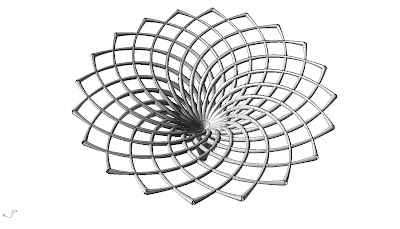## 24 April 2009

### parametrically...image credits: David Rutten

Interesting discussions about the definition of Parametric:

An example of massive parametric modelling at DRL (Design Research Laboratory, AA) thesis form third and final phase of parametric urbanism agenda: denCity is a Masters level research project by Sahra (Peter Sovinc, Saif Almasri, Suryansh Chandra)

## 15 April 2009

### Simple Honeycomb03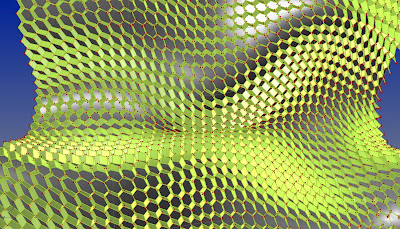Now with this grasshopper definition you can extrude the honeycomb lines radially normal to the surface, control extrusion lenght and U,V of surface.